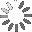# Example sentences for: per-household

How can you use “per-household” in a sentence? Here are some example sentences to help you improve your vocabulary:

• They are identical to Tables 1, 2 and 3 respectively, except that the total annual volumes in Tables 1, 2 and 3 have been replaced with per-household annual volumes in Tables 4, 5 and 6. A comparison of figures in Tables 4, 5, and 6 with the corresponding figures in Tables 1, 2 and 3, respectively, shows that the replacement of total by per-household volume figures leaves the shares of sectors and uses in total First-Class Mail volume unchanged.

• However, because the number of households has been increasing every year, the replacement of total by per-household volume figures produces annual growth rates which are lower than those shown in Tables 1, 2 and 3. That is, the positive annual growth rates in Tables 1, 2 and 3 shrink in Tables 4, 5 and 6 and the negative rates augment in absolute terms.

• Tables 4, 5 and 6 display First-Class per-household annual volumes.

• For example, if we compare the annual growth rates of mail volume for period 1993-97 shown in Column (11) of Tables 1 and 4 we can see that: (a) the 2.0 percent annual decrease of total HH-to-HH volume in Table 1 has been augmented to a 3.3 percent annual decrease of per-household volume in Table 4; (b) the 6.8 percent annual decrease of total HH-to-NHH volume in Table 1 has been augmented to a 8.0 percent annual decrease of per-household volume in Table 4; (c) the 0.2 percent annual increase of total NHH-to-HH volume in Table 1 has shrunk to a 1.2 percent annual decrease of per-household volume in Table 4; and (d) the 7.1 percent annual increase of total NHH-to-NHH volume in Table 1 has shrunk to a 5.7 percent annual increase of per-household volume in Table 4.

• Similarly, a comparison of annual growth rates for the same period in Table 2 with those in Table 5 reveals the following: (a) the 2.0 percent annual decrease of bill/payment volume in Table 2 has been augmented to a 3.3 percent annual decrease of perhousehold volume in Table 5; and (b) the 3.3 percent annual increase of total advertising mail volume in Table 2 has shrunk to a 1.8 percent annual increase of per-household volume in Table 5.

## Search

Search for example sentencesLoading...